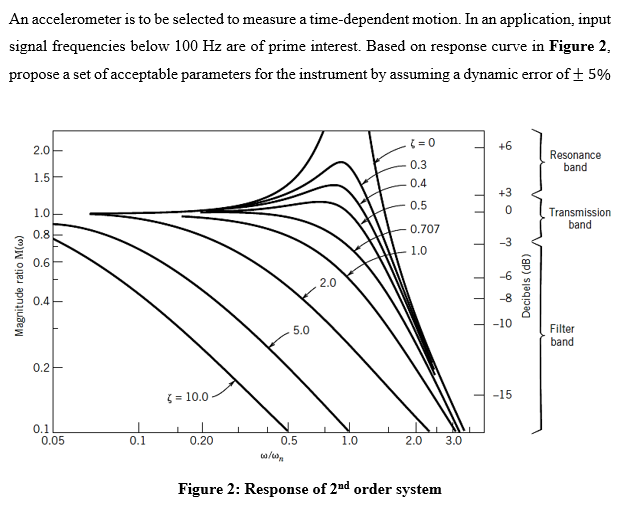# Question An accelerometer is to be selected to measure a time-dependent motion. In an application, input signal frequencies below 100 Hz are of prime interest. Based on response curve in Figure 2, propose a set of acceptable parameters for the instrument by assuming a dynamic error of + 5% ( = 0 +6 2.0 Resonance band 1.5 0.3 0.4 0.5 +3 0 1.0 Transmission band 0.707 0.8 -3 1.0 0.6 -6 Magnitude ratio Mw) 2.0 Decibels (dB) 0.4 -8 -10 5.0 Filter band 0.2 ( = 10.0 -15 0.1 0.05 0.1 0.20 1.0 2.0 3.0 0.5 co/, Figure 2: Response of 2nd order systemYY2NLQ The Asker · Mechanical EngineeringTranscribed Image Text: An accelerometer is to be selected to measure a time-dependent motion. In an application, input signal frequencies below 100 Hz are of prime interest. Based on response curve in Figure 2, propose a set of acceptable parameters for the instrument by assuming a dynamic error of + 5% ( = 0 +6 2.0 Resonance band 1.5 0.3 0.4 0.5 +3 0 1.0 Transmission band 0.707 0.8 -3 1.0 0.6 -6 Magnitude ratio Mw) 2.0 Decibels (dB) 0.4 -8 -10 5.0 Filter band 0.2 ( = 10.0 -15 0.1 0.05 0.1 0.20 1.0 2.0 3.0 0.5 co/, Figure 2: Response of 2nd order system
More
Transcribed Image Text: An accelerometer is to be selected to measure a time-dependent motion. In an application, input signal frequencies below 100 Hz are of prime interest. Based on response curve in Figure 2, propose a set of acceptable parameters for the instrument by assuming a dynamic error of + 5% ( = 0 +6 2.0 Resonance band 1.5 0.3 0.4 0.5 +3 0 1.0 Transmission band 0.707 0.8 -3 1.0 0.6 -6 Magnitude ratio Mw) 2.0 Decibels (dB) 0.4 -8 -10 5.0 Filter band 0.2 ( = 10.0 -15 0.1 0.05 0.1 0.20 1.0 2.0 3.0 0.5 co/, Figure 2: Response of 2nd order system